Win up to 100% scholarship on Aakash BYJU'S JEE/NEET courses with ABNAT Win up to 100% scholarship on Aakash BYJU'S JEE/NEET courses with ABNAT

# JEE Main 2022 June 29 – Shift 2 Physics Question Paper with Solutions

JEE is an entrance examination conducted for admission to different colleges across the nation. The JEE questions are based on Class 11 and Class 12 curricula. All of the answers to the questions from the JEE Main 2022 June 29 – Shift 2 Physics Question Paper with Solutions may be found on this page, for the reference of the candidates. The subject experts at BYJU’S have provided these solutions in a detailed manner.

## JEE Main 2022 June 29th Evening Shift Physics Question Paper and Solutions

#### SECTION – A

Multiple Choice Questions: This section contains 20 multiple choice questions. Each question has 4 choices (1), (2), (3) and (4), out of which ONLY ONE is correct.

1. A small toy starts moving from the position of rest under a constant acceleration. If it travels a distance of 10 m in t s, the distance travelled by the toy in the next t s will be :

(A) 10 m

(B) 20 m

(C) 30 m

(D) 40 m

Sol.

$$\begin{array}{l}\frac{1}{2} a t^{2}=10 \mathrm{~m}\end{array}$$
$$\begin{array}{l}\frac{1}{2} a(2 t)^{2}=40 \mathrm{~m}\end{array}$$

⇒ Distance travelled in next t s

= 40 – 10 = 30 m

2. At what temperature a gold ring of diameter 6.230 cm be heated so that it can be fitted on a wooden bangle of diameter 6.241 cm? Both the diameters have been measured at room temperature (27°C).

(Given: coefficient of linear thermal expansion of gold αL = 1.4 × 10–5 K–1)

(A) 125.7°C

(B) 91.7°C

(C) 425.7°C

(D) 152.7°C

Sol. ΔD = DαΔT

$$\begin{array}{l}\Delta T=\frac{0.011}{6.230 \times 1.4 \times 10^{-5}}\end{array}$$

= 126.11°C

Tf = T + ΔT

= (27 + 126.11)°C

= 153.11°C

⇒ Option (D) is correct

3. Two point charges Q each are placed at a distance d apart. A third point charge q is placed at a distance x from mid-point on the perpendicular bisector. The value of x at which charge q will experience the maximum Coulombs force is :

(A) x = d

$$\begin{array}{l}(B)\ x=\frac{d}{2}\end{array}$$
$$\begin{array}{l}(C)\ x=\frac{d}{\sqrt{2}}\end{array}$$
$$\begin{array}{l}(D)\ x=\frac{d}{2 \sqrt{2}}\end{array}$$

Sol. Force experienced by the charge q

$$\begin{array}{l}F=\frac{k Q q x}{\left[\left(\frac{d}{2}\right)^{2}+x^{2}\right]^{\frac{3}{2}}}\end{array}$$

For maximum Coulomb’s force for x

$$\begin{array}{l}\frac{d F}{d x}=0\end{array}$$

On solving

$$\begin{array}{l}x=\frac{d}{2 \sqrt{2}}\end{array}$$

4. The speed of light in media ‘A’ and ‘B’ are 2.0 × 1010 cm/s and 1.5 × 1010 cm/s respectively. A ray of light enters from the medium B to A at an incident angle ‘θ’. If the ray suffers total internal reflection, then

$$\begin{array}{l}(A)\ \theta=\sin ^{-1}\left(\frac{3}{4}\right)\end{array}$$
$$\begin{array}{l}(B)\ \theta>\sin ^{-1}\left(\frac{2}{3}\right)\end{array}$$
$$\begin{array}{l}(C)\ \theta<\sin ^{-1}\left(\frac{3}{4}\right)\end{array}$$
$$\begin{array}{l}(D)\ \theta>\sin ^{-1}\left(\frac{3}{4}\right)\end{array}$$

Sol.

$$\begin{array}{l}\mu_{A}=\frac{3 \times 10^{8}}{2 \times 10^{8}}=1.5\end{array}$$
$$\begin{array}{l}\mu_{B}=\frac{3 \times 10^{8}}{1.5 \times 10^{8}}=2\end{array}$$

For TIR

θ > ic

$$\begin{array}{l}\theta>\sin ^{-1}\left(\frac{1.5}{2}\right)\end{array}$$
$$\begin{array}{l}\theta>\sin ^{-1}\left(\frac{3}{4}\right)\end{array}$$

5. In the following nuclear reaction,

$$\begin{array}{l}D \stackrel{\alpha}{\longrightarrow} D_{1} \stackrel{\beta^{-}}{\longrightarrow} D_{2} \stackrel{\alpha}{\longrightarrow} D_{3} \stackrel{\gamma}{\longrightarrow} D_{4}\end{array}$$
Mass number of D is 182 and atomic number is 74. Mass number and atomic number of D4, respectively, will be _____.

(A) 174 and 71

(B) 174 and 69

(C) 172 and 69

(D) 172 and 71

Sol. Equivalent reaction can be written as

DD4 + 2α + β + γ

⇒ Mass number of D4 = Mass number of D – 2 × 4

= 182 – 8 = 174

⇒ Atomic number of D4

= Atomic number of D – 2 × 2 + 1

= 74 – 4 + 1 = 71

6. The electric field at a point associated with a light wave is given by

E = 200[sin(6 × 1015)t + sin(9 × 1015)t] Vm–1

Given : h = 4.14 × 10–15 eVs

If this light falls on a metal surface having a work function of 2.50 eV, the maximum kinetic energy of the photoelectrons will be

(A) 1.90 eV

(B) 3.27 eV

(C) 3.60 eV

(D) 3.42 eV

Sol. Frequency of EM waves

$$\begin{array}{l}=\frac{6}{2 \pi} \times 10^{15}\end{array}$$
and
$$\begin{array}{l}\frac{9}{2 \pi} \times 10^{15}\end{array}$$

Energy of one photon of these waves

$$\begin{array}{l}=\left(4.14 \times 10^{-15} \times \frac{6}{2 \pi} \times 10^{15}\right) \mathrm{eV}\end{array}$$

and

$$\begin{array}{l}\left(4.14 \times 10^{-15} \times \frac{9}{2 \pi} \times 10^{15}\right) \mathrm{eV}\end{array}$$

= 3.95 eV and 5.93 eV

⇒ Energy of maximum energetic electrons

= 5.93 – 2.50 = 3.43 eV

7. A capacitor is discharging through a resistor R. Consider in time t1, the energy stored in the capacitor reduces to half of its initial value and in time t2, the charge stored reduces to one eighth of its initial value. The ratio t1/t2 will be

$$\begin{array}{l}(A)\ \frac{1}{2}\end{array}$$
$$\begin{array}{l}(B)\ \frac{1}{3}\end{array}$$
$$\begin{array}{l}(C)\ \frac{1}{4}\end{array}$$
$$\begin{array}{l}(D)\ \frac{1}{6}\end{array}$$

Sol. For a discharging capacitor when energy reduces to half the charge would become 1/√2 times the initial value.

$$\begin{array}{l}\Rightarrow\left(\frac{1}{2}\right)^{1 / 2}=e^{-t_{1} / \tau}\end{array}$$

Similarly,

$$\begin{array}{l}\left(\frac{1}{2}\right)^{3}=e^{-t_{2}/\tau}\end{array}$$
$$\begin{array}{l}\Rightarrow \frac{t_{1}}{t_{2}}=\frac{1}{6}\end{array}$$

8. Starting with the same initial conditions, an ideal gas expands from volume V1 to V2 in three different ways. The work done by the gas is W1 if the process is purely isothermal, W2, if the process is purely adiabatic and W3 if the process is purely isobaric. Then, choose the correct option.

(A) W1 < W2 < W3

(B) W2 < W3 < W1

(C) W3 < W1 < W2

(D) W2 < W1 < W3

Sol.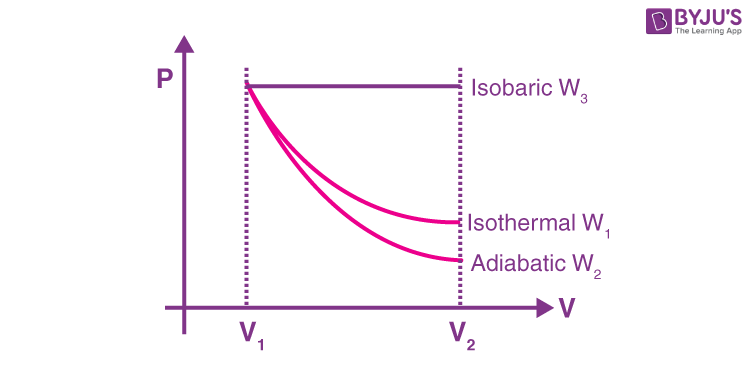Comparing the area under the PV graph

A3 > A1 > A2

W3 > W1 > W2

9. Two long current carrying conductors are placed parallel to each other at a distance of 8 cm between them. The magnitude of magnetic field produced at mid-point between the two conductors due to current flowing in them is 30 μT. The equal current flowing in the two conductors is:

(A) 30 A in the same direction

(B) 30 A in the opposite direction

(C) 60 A in the opposite direction

(D) 300 A in the opposite direction

Sol. As Bnet ≠ 0 that is the wires are carrying current in opposite direction.

$$\begin{array}{l}\frac{\mu_{0} l \times 2}{2 \pi\left(4 \times 10^{-2}\right)}=30 \times 10^{-6} \mathrm{~T} \end{array}$$
$$\begin{array}{l}\Rightarrow I=\frac{30 \times 10^{-6}}{10^{-6}} \mathrm{~A}=30 \mathrm{~A}\end{array}$$
in opposite direction.

10. The time period of a satellite revolving around earth in a given orbit is 7 hours. If the radius of orbit is increased to three times its previous value, then approximate new time period of the satellite will be

(A) 40 hours

(B) 36 hours

(C) 30 hours

(D) 25 hours

Sol.

$$\begin{array}{l}T_{2}^{2}=\left(\frac{R_{2}}{R_{1}}\right)^{3} T_{1}^{2} \end{array}$$
$$\begin{array}{l}\Rightarrow T_{2} =(3)^{3 / 2} \times 7 \approx 5.2 \times 7 \end{array}$$
$$\begin{array}{l}T_{2} \cong 36\ \mathrm{hrs}\end{array}$$

11. The TV transmission tower at a particular station has a height of 125 m. For doubling the coverage of its range, the height of the tower should be increased by

(A) 125 m

(B) 250 m

(C) 375 m

(D) 500 m

Sol. Range

$$\begin{array}{l}R=\sqrt{2 h \mathrm{Re}}\end{array}$$

Let the height be h′ to double the range so

$$\begin{array}{l}2 R=\sqrt{2 h^{\prime} \mathrm{Re}}\end{array}$$

On solving h′ = 4h

h′ = 500 m

So Δh = 375 m

12. The motion of a simple pendulum executing S.H.M. is represented by the following equation. y = A sin(πt + φ), where time is measured in second. The length of pendulum is

(A) 97.23 cm

(B) 25.3 cm

(C) 99.4 cm

(D) 406.1 cm

Sol.

$$\begin{array}{l}\omega=\pi=\sqrt{\frac{g}{\ell}}\end{array}$$

So

$$\begin{array}{l}\ell =\frac{g}{\pi^{2}}\end{array}$$

≃ 99.4 cm (Nearest value)

13. A vessel contains 16 g of hydrogen and 128 g of oxygen at standard temperature and pressure. The volume of the vessel in cm3 is:

(A) 72 × 105

(B) 32 × 105

(C) 27 × 104

(D) 54 × 104

Sol. Total number of moles are

\begin{array}{l}\begin{aligned}n &=n_{\mathrm{H}_{2}}+n_{\mathrm{O}_{2}} \\&=\frac{16}{2}+\frac{128}{32} \\&=12 \text { moles }\end{aligned}\end{array}

Using PV = nRT

$$\begin{array}{l}V =\frac{n R T}{P}\end{array}$$
$$\begin{array}{l}=\frac{12 \times 8.31 \times 273.15}{10^{5}} \mathrm{~m}^{3}\end{array}$$

= 0.27 m3 = 27 × 104 cm3

14. Given below are two statements:

Statement I: The electric force changes the speed of the charged particle and hence changes its kinetic energy; whereas the magnetic force does not change the kinetic energy of the charged particle.

Statement II: The electric force accelerates the positively charged particle perpendicular to the direction of electric field. The magnetic force accelerates the moving charged particle along the direction of magnetic field.

In the light of the above statements, choose the most appropriate answer from the options given below:

(A) Both statement I and statement II are correct

(B) Both statement I and statement II are incorrect

(C) Statement I is correct but statement II is incorrect

(D) Statement I is incorrect but statement II is correct

Sol. Electric field accelerates the particle in the direction of field

$$\begin{array}{l}(\vec{F}=q \vec{E}=m \vec{a})\end{array}$$
and magnetic field accelerates the particle perpendicular to the field
$$\begin{array}{l}(\vec{F}=q \vec{v} \times \vec{B}=m \vec{a})\end{array}$$

15. A block of mass 40 kg slides over a surface, when a mass of 4 kg is suspended through an inextensible massless string passing over frictionless pulley as shown below.

The coefficient of kinetic friction between the surface and block is 0.02. The acceleration of block is (Given g = 10 ms–2.)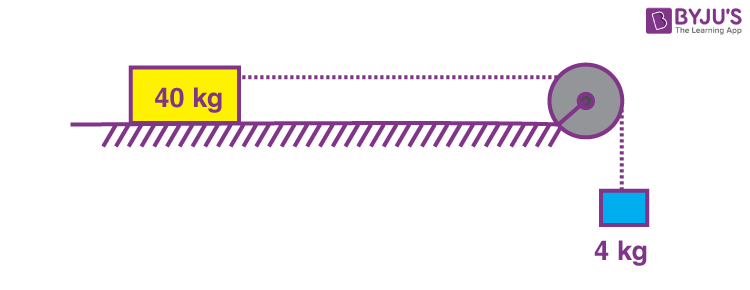(A) 1 ms–2

(B) 1/5 ms–2

(C) 4/5 ms–2

(D) 8/11 ms–2

Sol.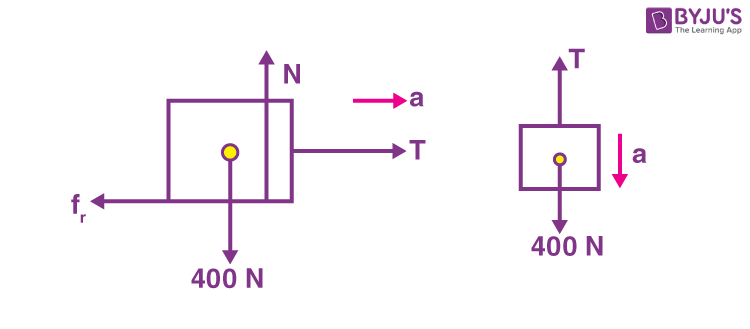= 0.02 × 400

= 8 N

Let the acceleration is a as shown then.

40 – T = 4a

T – 8 = 40a

$$\begin{array}{l}\Rightarrow \quad a=\frac{32}{44}=\frac{8}{11} \mathrm{~m} / \mathrm{s}^{2}\end{array}$$

16. In the given figure, the block of mass m is dropped from the point ‘A’. The expression for kinetic energy of block when it reaches point ‘B’ is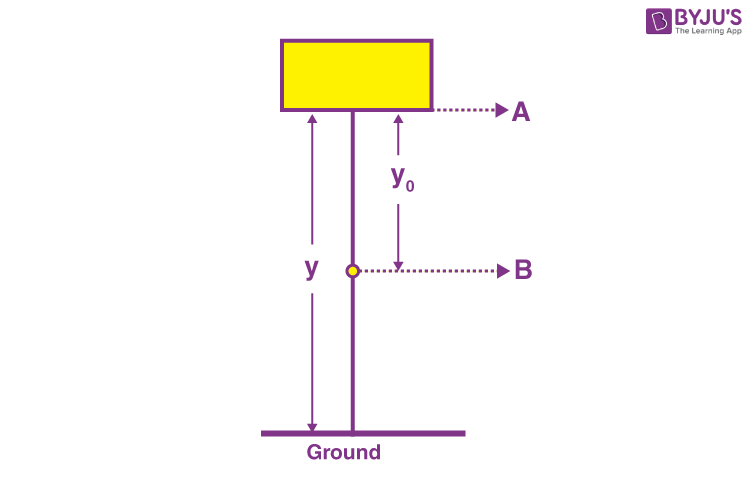$$\begin{array}{l}(A)\ \frac{1}{2} m g y_{0}^{2}\end{array}$$
$$\begin{array}{l}(B)\ \frac{1}{2} m g y^{2}\end{array}$$

(C) mg(yy0)

(D) mgy0

Sol. Loss is potential energy = gain in kinetic energy

– (mg(yy0) – mgy) = KE – 0

KE = mgy0

17. A block of mass M placed inside a box descends vertically with acceleration ‘a’. The block exerts a force equal to one-fourth of its weight on the floor of the box.

The value of ‘a’ will be

$$\begin{array}{l}(A)\ \frac{g}{4}\end{array}$$
$$\begin{array}{l}(B)\ \frac{g}{2}\end{array}$$
$$\begin{array}{l}(C)\ \frac{3g}{4}\end{array}$$

(D) g

Sol.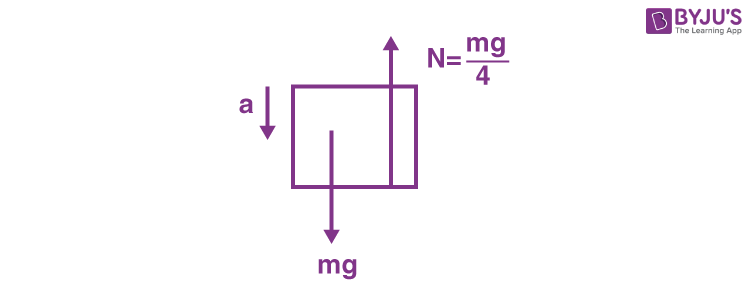Using Newton’s second law

$$\begin{array}{l}m g-\frac{m g}{4}=ma\end{array}$$
$$\begin{array}{l}\Rightarrow a=\frac{3 g}{4}\end{array}$$

18. If the electric potential at any point (x, y, z)m in space is given by V = 3x2 volt. The electric field at the point (1, 0, 3)m will be

(A) 3 Vm–1, directed along positive x-axis

(B) 3 Vm–1, directed along negative x-axis

(C) 6 Vm–1, directed along positive x-axis

(D) 6 Vm–1, directed along negative x-axis

Sol.

$$\begin{array}{l}\vec{E}=-\frac{d V}{d x} \hat{i}\end{array}$$
$$\begin{array}{l}\vec{E}=-6 x \hat{i}\end{array}$$

So,

$$\begin{array}{l}\vec{E}\ \text{at}\ (1, 0, 3)\ \text{is}\end{array}$$
$$\begin{array}{l}\vec{E}=-6 \hat{i} \quad \mathrm{~V} / \mathrm{m}\end{array}$$

19. The combination of two identical cells, whether connected in series or parallel combination provides the same current through an external resistance of 2 Ω. The value of internal resistance of each cell is

(A) 2 Ω

(B) 4 Ω

(C) 6 Ω

(D) 8 Ω

Sol.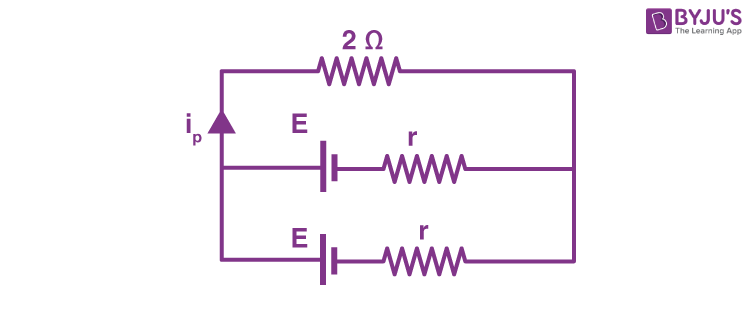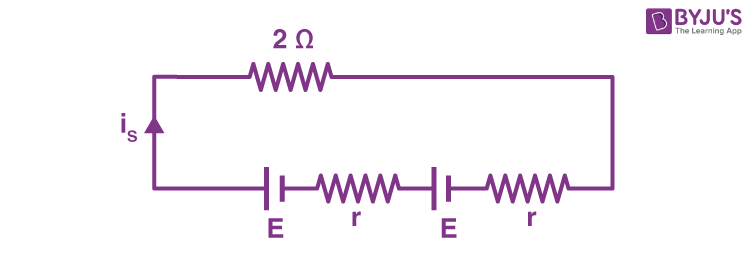From diagram

$$\begin{array}{l}i_{p}=\frac{E}{2+\frac{r}{2}} \text { and } i_{s}=\frac{2 E}{2+2 r}\end{array}$$

given ip = is

$$\begin{array}{l}\frac{1}{2+\frac{r}{2}}=\frac{1}{1+r}\end{array}$$
$$\begin{array}{l}1+r=2+\frac{r}{2}\end{array}$$
$$\begin{array}{l}r=2~\Omega\end{array}$$

20. A person can throw a ball upto a maximum range of 100 m. How high above the ground he can throw the same ball?

(A) 25 m

(B) 50 m

(C) 100 m

(D) 200 m

Sol.

$$\begin{array}{l}R_{\max }=\frac{u^{2}}{g}=100 \mathrm{~m}\end{array}$$

So,

$$\begin{array}{l}H_{\max }=\frac{u^{2}}{2 g}=50 \mathrm{~m}\end{array}$$

#### SECTION – B

Numerical Value Type Questions: This section contains 10 questions. In Section B, attempt any five questions out of 10. The answer to each question is a NUMERICAL VALUE. For each question, enter the correct numerical value (in decimal notation, truncated/rounded-off to the second decimal place; e.g. 06.25, 07.00, –00.33, –00.30, 30.27, –27.30) using the mouse and the on-screen virtual numeric keypad in the place designated to enter the answer.

1. The vernier constant of Vernier callipers is 0.1 mm and it has zero error of (–0.05) cm. While measuring diameter of a sphere, the main scale reading is 1.7 cm and coinciding vernier division is 5. The corrected diameter will be ________× 10–2 cm.

Sol. Since zero error is negative, we will add 0.05 cm.

⇒ Corrected reading = 1.7 cm + 5 × 0.1 mm + 0.05 cm

= 180 × 10–2 cm

2. A small spherical ball of radius 0.1 mm and density 104 kg m–3 falls freely under gravity through a distance h before entering a tank of water. If, after entering the water the velocity of ball does not change and it continue to fall with same constant velocity inside water, then the value of h will be ______ m.

(Given g = 10 ms–2, viscosity of water = 1.0 × 10–5 N-sm–2).

Sol.

$$\begin{array}{l}\sqrt{2 g h} = \text{terminal speed}\end{array}$$
$$\begin{array}{l}\Rightarrow \sqrt{2 g h} =\frac{2}{9} \frac{r^{2} g\left(\rho-\rho^{\prime}\right)}{\eta}\end{array}$$
$$\begin{array}{l}=\frac{2}{9} \times \frac{10^{-8} \times 10 \times 9000}{10^{-5}}\end{array}$$
$$\begin{array}{l}\Rightarrow h=\frac{400}{2 g}\end{array}$$

h = 20 m

3. In an experiment to determine the velocity of sound in air at room temperature using a resonance tube, the first resonance is observed when the air column has a length of 20.0 cm for a tuning fork of frequency 400 Hz is used. The velocity of the sound at room temperature is 336 ms–1. The third resonance is observed when the air column has a length of ______ cm.

Sol.

$$\begin{array}{l}400=\frac{v}{4\left(L_{1}+e\right)}…(i)\end{array}$$
$$\begin{array}{l}400=\frac{5 v}{4\left(L_{2}+e\right)}…(ii)\end{array}$$
$$\begin{array}{l}\Rightarrow L_{1}+e=\frac{\lambda}{4}=21 \mathrm{~cm}\end{array}$$
$$\begin{array}{l}L_{2}+e=\frac{5 \lambda}{4}=105 \mathrm{~cm}\end{array}$$

e = 1 cm & L2 = 104 cm

4. Two resistors are connected in series across a battery as shown in figure. If a voltmeter of resistance 2000 Ω is used to measure the potential difference across 500 Ω resistor, the reading of the voltmeter will be _____ V.Sol. New

$$\begin{array}{l}R_{\text {eff }}=\frac{2000 \times 500}{2500}+600~ \Omega=1000 ~\Omega\end{array}$$

$$\begin{array}{l}=\frac{400}{1000} \times 20=8 \text { volts }\end{array}$$

5. A potential barrier of 0.4 V exists across a p-n junction. An electron enters the junction from the n-side with a speed of 6.0 × 105 ms–1. The speed with which electrons enters the p side will be

$$\begin{array}{l}\frac{x}{3} \times 10^5 ~\text{ms}^{-1}\end{array}$$
the value of x is _________.

(Give mass of electron = 9 × 10–31 kg, charge on electron = 1.6 × 10–19 C)

Sol. Conserving energy,

$$\begin{array}{l}\frac{1}{2} m v^{2}=\frac{1}{2} m\left(6 \times 10^{5}\right)^{2}-0.4 \mathrm{eV}\end{array}$$
$$\begin{array}{l}\Rightarrow v=\sqrt{\left(6 \times 10^{5}\right)^{2}-\frac{2 \times 1.6 \times 10^{-19} \times 0.4}{9 \times 10^{-31}}}\end{array}$$
\begin{array}{l}\begin{aligned}&=\sqrt{36 \times 10^{10}-\frac{1.28}{9}\times 10^{12}} \\ \Rightarrow v&=\frac{14}{3} \times 10^{5} \mathrm{~m} / \mathrm{s} \\ \Rightarrow x&=14\end{aligned}\end{array}

6. The displacement current of 4.425 μA is developed in the space between the plates of parallel plate capacitor when voltage is changing at a rate of 106 Vs–1. The area of each plate of the capacitor is 40 cm2. The distance between each plate of the capacitor x × 10–3 m. The value of x is,

(Permittivity of free space, E0 = 8.85 × 10–12 C2 N–1 m–2)

Sol.

$$\begin{array}{l} 4.425 \mu\text{ A } =\frac{E_{0} A}{d} \times \frac{d V}{d t}\end{array}$$

$$\begin{array}{l}d=\frac{8.85 \times 10^{-12} \times 40 \times 10^{-4}}{4.425 \times 10^{-6}} \times 10^{6} \end{array}$$

d = 8 × 10–3 m

x = 8

7. The moment of inertia of a uniform thin rod about a perpendicular axis passing through one end is I1. The same rod is bent into a ring and its moment of inertia about a diameter is I2. If

$$\begin{array}{l}\frac{I_{1}}{I_{2}}\ is\ \frac{x \pi^{2}}{3},\end{array}$$
then the value of x will be ______.

Sol.

$$\begin{array}{l}I_{1}=\frac{M L^{2}}{3}….(1)\end{array}$$

For ring :

$$\begin{array}{l}I_{2}=\frac{M R^{2}}{2}\end{array}$$

and 2πR = L

$$\begin{array}{l}\Rightarrow I_{2}=\frac{M}{2}\left(\frac{L^{2}}{4 \pi^{2}}\right)….(2)\end{array}$$
$$\begin{array}{l}\Rightarrow \frac{I_{1}}{I_{2}}=\frac{8 \pi^{2}}{3}\end{array}$$

x = 8

8. The half life of a radioactive substance is 5 years. After x years, a given sample of the radioactive substance gets reduced to 6.25% of its initial value. The value of x is ______.

Sol. N = N0e–λt

$$\begin{array}{l}\Rightarrow \frac{6.25}{100}=e^{-\lambda t}\end{array}$$
$$\begin{array}{l}\Rightarrow e^{-\lambda t}=\frac{1}{16}=\left(\frac{1}{2}\right)^{4}\end{array}$$

t = 4t1/2

t = 20 years

9. In a double slit experiment with monochromatic light, fringes are obtained on a screen placed at some distance from the plane of slits. If the screen is moved by 5 × 10–2 m towards the slits, the change in fringe width is 3 × 10–3 cm. If the distance between the slits is 1 mm, then the wavelength of the light will be _______ nm.

Sol. Fringe width

$$\begin{array}{l}\beta=\frac{\lambda D}{d}\end{array}$$
$$\begin{array}{l}\Rightarrow |d \beta|=\frac{\lambda}{d}|d(D)|\end{array}$$
$$\begin{array}{l}\Rightarrow 3 \times 10^{-3} \mathrm{~cm}=\frac{\lambda}{1 \mathrm{~mm}}\left(5 \times 10^{-2} \mathrm{~m}\right)\end{array}$$
$$\begin{array}{l}\Rightarrow \lambda=\frac{3 \times 10^{-8}}{5 \times 10^{-2}} \mathrm{~m}\end{array}$$

⇒ λ = 600 nm

10. An inductor of 0.5 mH, a capacitor of 200 μF and a resistor of 2 Ω are connected in series with a 220 V ac source. If the current is in phase with the emf, the frequency of ac source will be ______ × 102 Hz.

Sol. Current will be in phase with emf when

$$\begin{array}{l}\omega L=\frac{1}{\omega C}\end{array}$$
$$\begin{array}{l}\Rightarrow \omega=\frac{1}{\sqrt{L C}}=\frac{1}{\sqrt{5 \times 10^{-4} \times 2 \times 10^{-4}}}\end{array}$$
$$\begin{array}{l}\Rightarrow \omega=\frac{10^{4}}{\sqrt{10}}~ \mathrm{rad} / \mathrm{s}\end{array}$$
$$\begin{array}{l}\Rightarrow f=\frac{1}{2 \pi} \times \frac{10^{4}}{\sqrt{10}} \mathrm{~Hz}\end{array}$$
$$\begin{array}{l}\Rightarrow f\simeq500 ~\text{Hz}\end{array}$$

### Download PDF of JEE Main 2022 June 29 Shift 2 Physics Paper & Solutions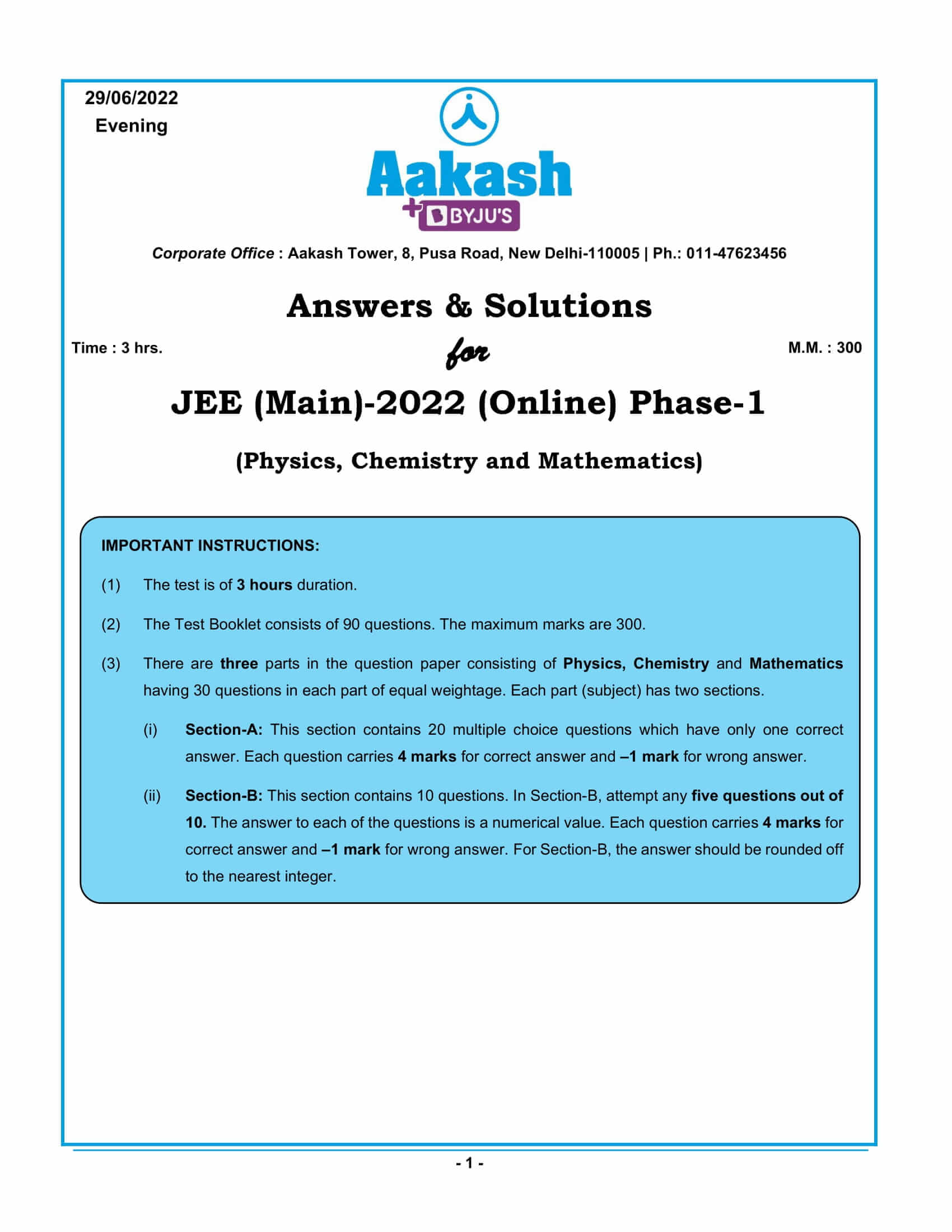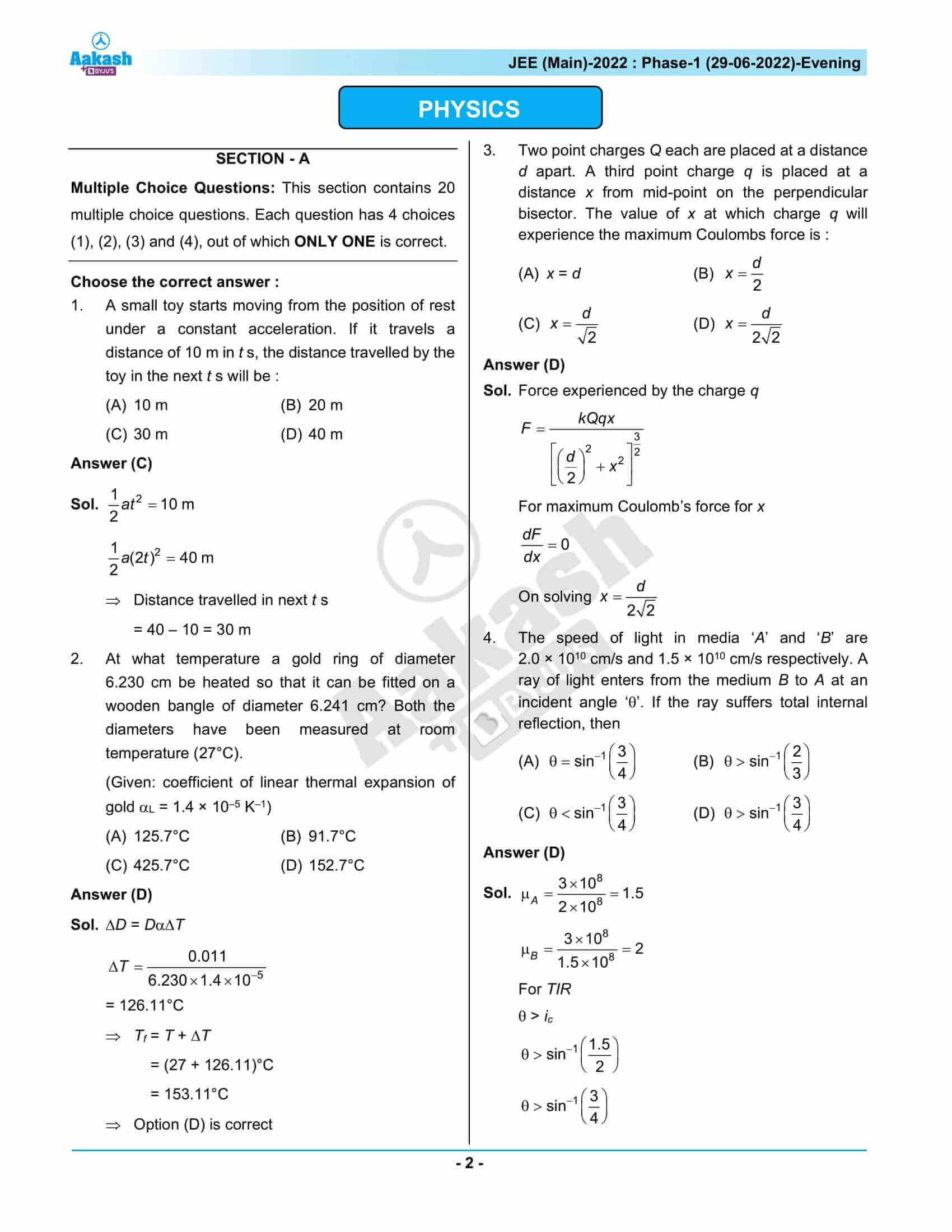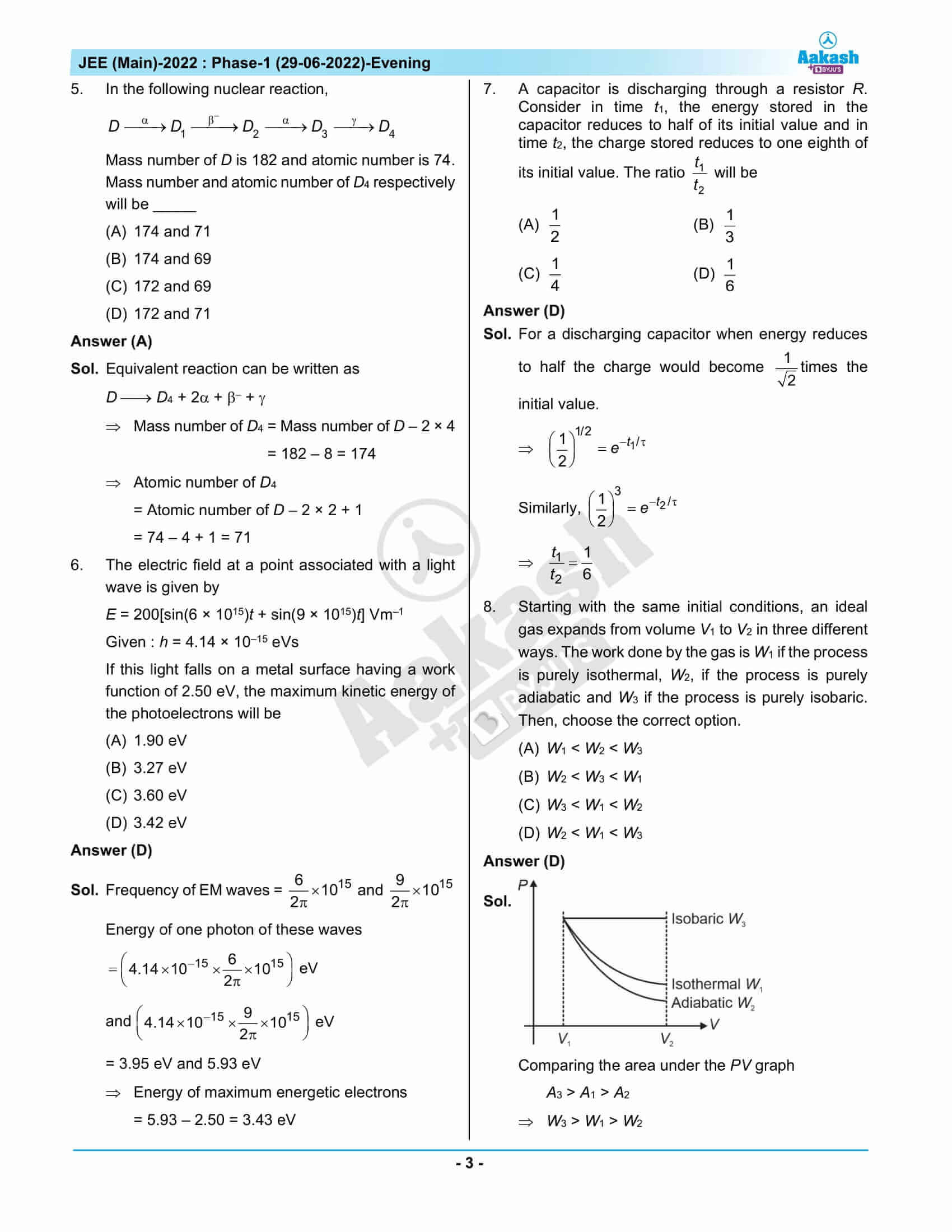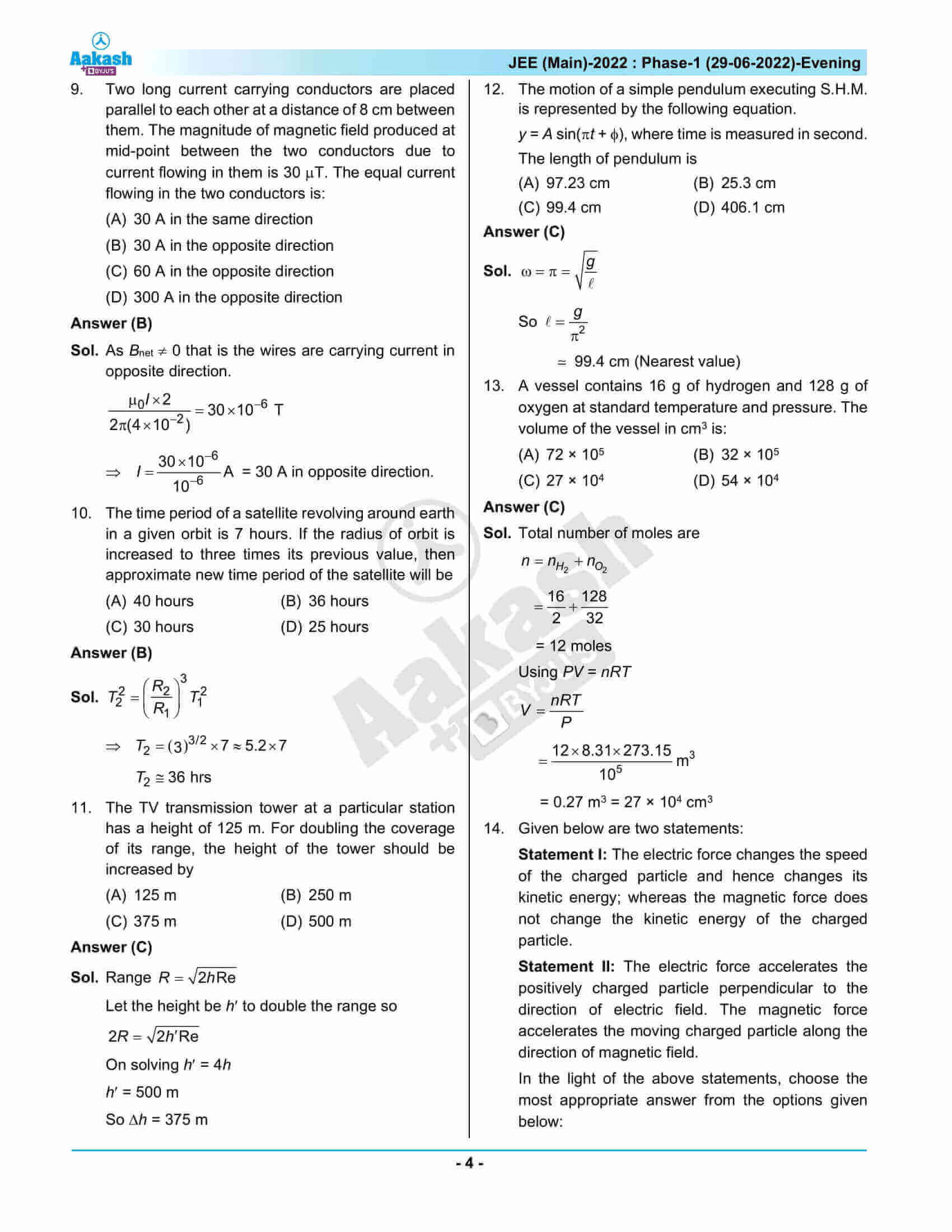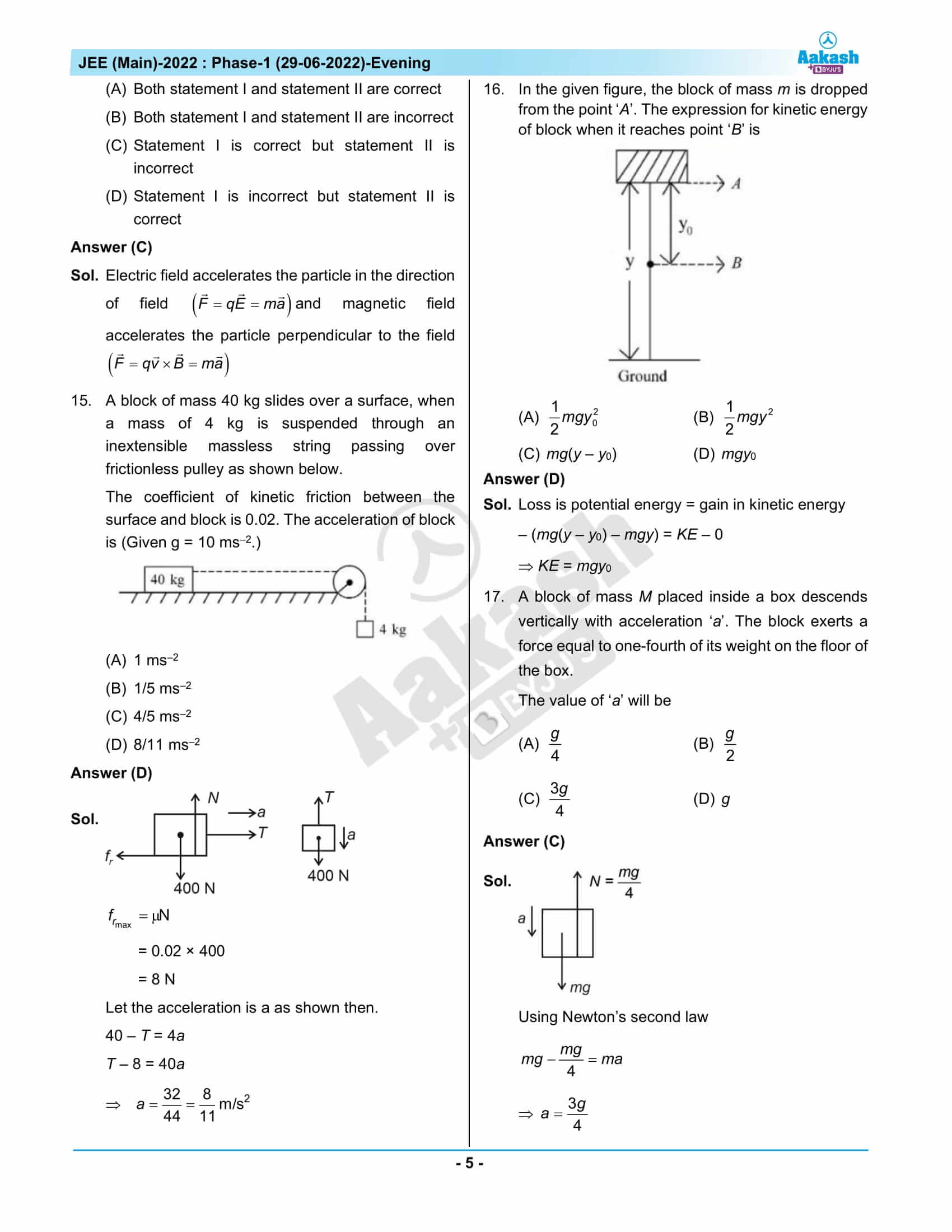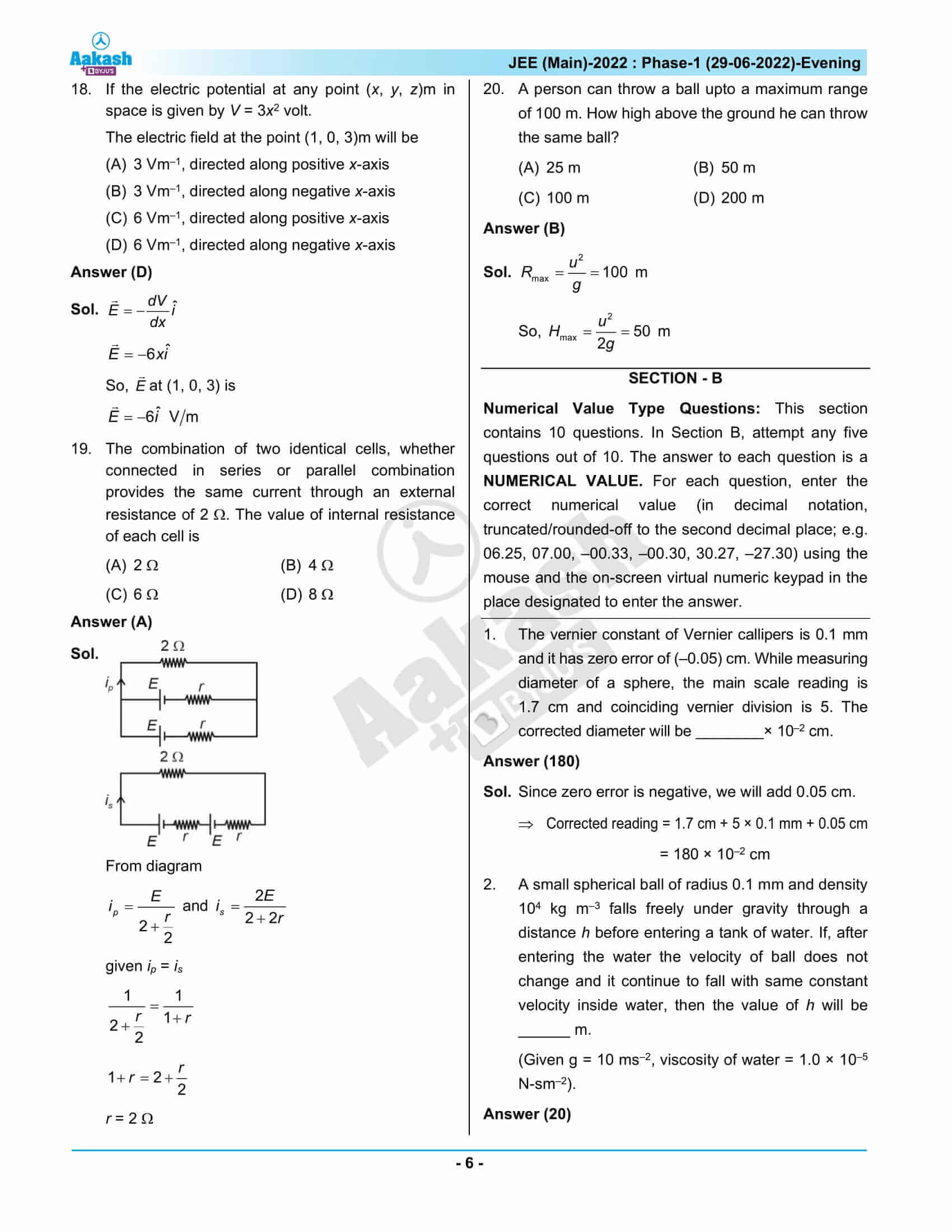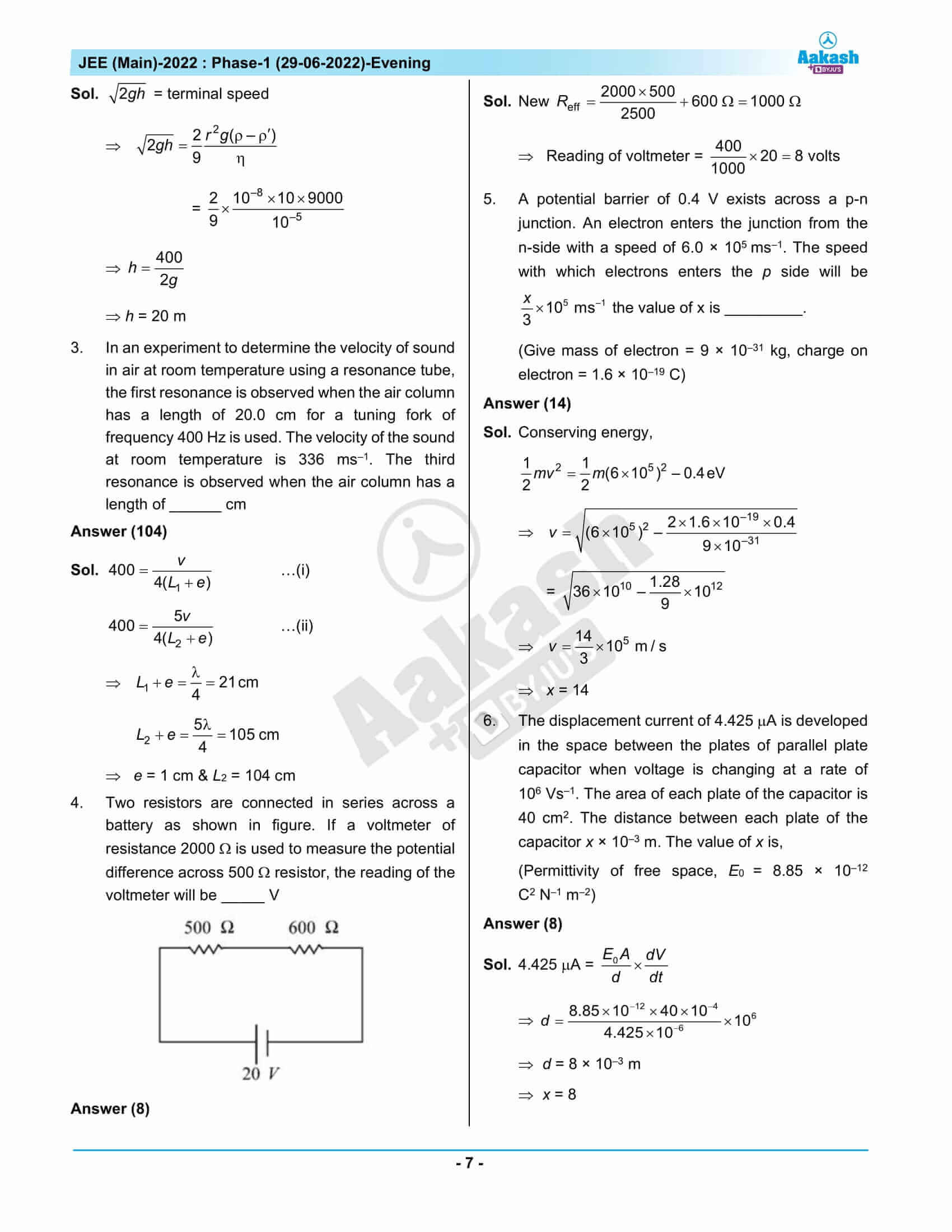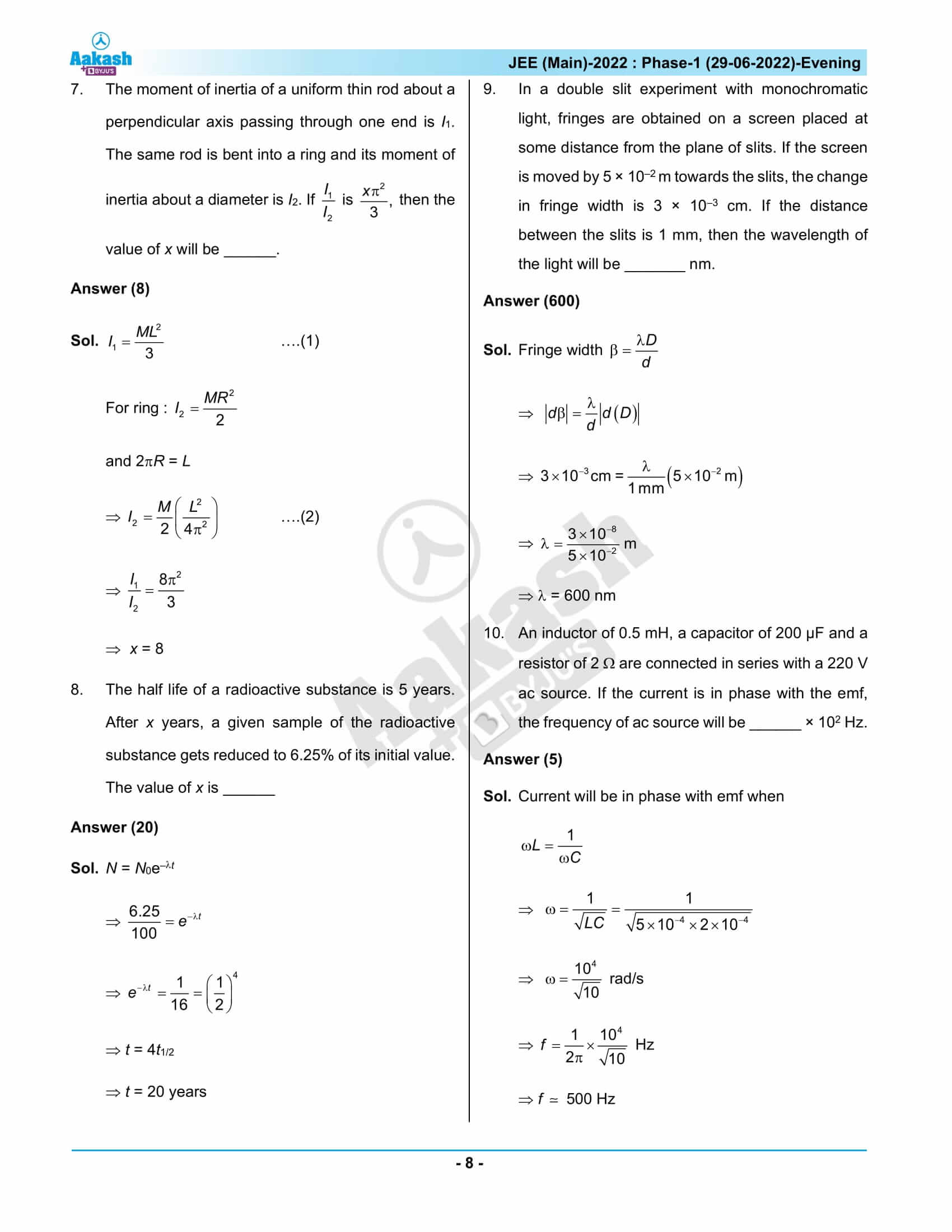## JEE Main 2022 June 29th Shift 2 Question Paper Analysis

### JEE Main 2022 June 29 Shift 2 Question Paper – Physics Solutions### JEE Main 2022 June 29 Shift 2 Question Paper – Chemistry Solutions### JEE Main 2022 June 29 Shift 2 Question Paper – Maths Solutions## Frequently Asked Questions – FAQs

Q1

### What type of questions were asked in JEE Main 2022 June 29 – Shift 2 Physics Question Paper?

The physics part of the question paper has sections A and B. Section A questions are multiple choice questions and section B questions are single answer types.

Q2

### How are the marks are allotted for the Physics section of JEE Main 2022 June 29 shift 2 question paper?

Section A of the Physics questions has 20 questions and section B has 10 questions. The candidates should answer all the questions from section A and only 5 questions from section B. Each question is awarded 4 marks. Therefore, a total of 80 marks are allotted for section A and 20 marks are allotted for section B.

Q3

### What are the Physics chapters included from the Class 11 syllabus in JEE Main 2022 June 29th second shift paper?

The Physics chapters included are:
Laws of Motion
Motion In A Plane
Motion In A Straight Line
Work, Energy and Power
Thermodynamics
Oscillations
System of Particles and Rotational Motion
Gravitation
Units and Measurements
Waves

Q4

### What was the class-wise question distribution of the Physics questions in JEE Main 2022 June 29 – Shift 2 Question Paper?

Of the 27 memory-based questions, 13 questions are from Class 11 and 14 questions are from Class 12.

Q5

### What is the difficulty level of the Physics questions in the JEE Main 2022 29th June shift 2 question paper?

The difficulty level of the Physics questions is rated 1.48 out of 3.